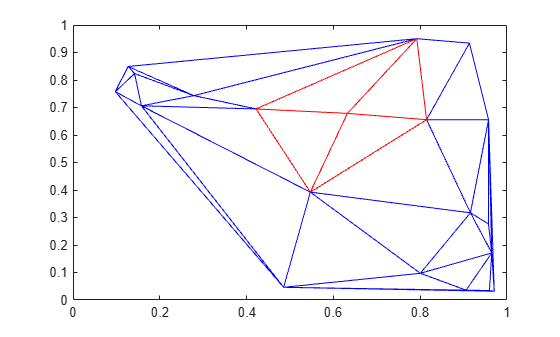vertexAttachments

Triangles or tetrahedra attached to vertex

Description

V = vertexAttachments(TR) returns the IDs of the triangles or tetrahedra attached to every vertex in the triangulation TR. A triangle or tetrahedron ID is the row number of the corresponding triangle or tetrahedron in the property TR.ConnectivityList.

The output v is an m-by-1 cell array where m is the number of vertices in TR. Each element of V contains the IDs of the attached triangles or tetrahedra corresponding to that vertex. The vertices can have a different number of attached triangles or tetrahedra depending on where they are located in the triangulation.

example

V = vertexAttachments(TR,ID) returns the IDs of the triangles or tetrahedra attached to the vertices specified in ID. The vertex IDs in ID are the row numbers of the corresponding vertices in the property TR.Points.

Examples

collapse all

Locate and plot the attachments to a specified vertex in a 2-D Delaunay triangulation.

Create a Delaunay triangulation from a set of 2-D points.

rng default;
x = rand([20,1]);
y = rand([20,1]);
TR = delaunayTriangulation(x,y);

Find the triangles attached to the 5th vertex.

V = vertexAttachments(TR,5);
V{:}
ans = 1×4

6     7    12    10

Plot the triangulation, and plot the triangles attached to vertex 5 in red.

triplot(TR)
hold on
triplot(TR(V{:},:),x,y,'Color','r')Input Arguments

collapse all

Triangulation representation, specified as a scalar triangulation or delaunayTriangulation object.

Data Types: triangulation | delaunayTriangulation

Vertex IDs of the query points, returned as a column vector. A vertex ID is the row number of the corresponding vertex in the Points property.

Data Types: double